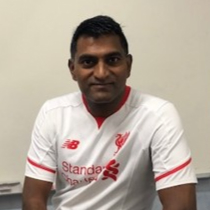# P2 Curriculum Maths Lessons 2nd Semester

###### Key Topics Covered in this Courses
• Numbers To 10
• Number Bonds
• Subtraction Within 10
• Shapes And Patterns
• Ordinal Numbers And Position
• Numbers To 20
• Addition And Subtraction Within 20
• Length
• Numbers To 40
###### Course Description

Mastering Singapore P2 curriculum maths at your own pace. Include a detailed explanation of hundreds of problems to let the learner grasp the basic concepts and methods of P2 mathematics. Video lessons with quizzes to check the progress.

3 months

##### Course Price

SGD 300.00 SGD 200.00

##### Course Description

For students from lower primary grades, it is essential to let them have this interest in mathematics. As it is the interest that drives them through the studies in mathematics in their future. When a child is interested in mathematics, it will let them study with much higher efficiency, and also tend to be spending more time and effort, thus resulting in better learning than everyone. For many students that hates maths, it is often caused by this vicious cycle: students are not very good at math→ students don’t like studying maths→ students are not willing to study math→ students are worse at math. Similarly, if we let the students be interested in math, it can go the other way, and the snowballing will continue until their success.

Mastering Singapore P2 curriculum maths at your own pace.
Include a detailed explanation of hundreds of problems to let the learner grasp the basic concepts and methods of P2 mathematics.
Video lessons with quizzes to check the progress.

Lessons are organised into the following topics:

• Numbers to 1000
• Subtraction within 1000
• Word Problems involving Addition and Subtraction
• Multiplication & Division
• Multiplication Table of 2 & 3
• Multiplication Table of 4, 5 & 10
• Word Problems involving Multiplication & Division##### Mr Bala
Master Trainer

Mr Bala has been teaching Math Olympiad for 20 years. He is dedicated, helpful and caring, making sure students gain a strong understanding of concepts. Students like him a lot and he has helped many students develop confidence and an interest in maths.